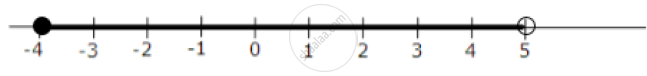Share

# Solve the Following Inequation and Represent the Solution Set on the Number Line: 4x - 19 < (3x)/5 - 2 <= (-2)/5 + X, X ∈ R - ICSE Class 10 - Mathematics

ConceptRepresentation of Solution on the Number Line

#### Question

Solve the following inequation and represent the solution set on the number line:

4x - 19 < (3x)/5 - 2 <= (-2)/5 + x, x ∈ R

#### Solution

The Given inequation is

4x - 19 < (3x)/5 - 2 <= (-2)/5 + x, x ∈ R

=> (4x - 19) < (3x - 10)/5 <= (-2 + 5x)/5

=> 5(4x - 19) < 3x - 10 < -2 + 5x

=>  20x - 95 < 3x - 10 <= -2 + 5x

Solving 20x - 95 < 3x - 10

=> 17x < 85

=> x < 5

Solving 3x - 10 <= -2 + 5x

=> -2x <= 8

=> -x <= 4

=> x >= -4

So the solution set = {x : -4 <= x < 5, x in R}

Representation on the Number lineIs there an error in this question or solution?

#### APPEARS IN

2011-2012 (March) (with solutions)
Question 6.2 | 3.00 marks
Solution Solve the Following Inequation and Represent the Solution Set on the Number Line: 4x - 19 < (3x)/5 - 2 <= (-2)/5 + X, X ∈ R Concept: Representation of Solution on the Number Line.
S